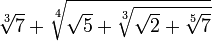# An Algebraic Number

(diff) ← Older revision | Latest revision (diff) | Newer revision → (diff)$\sqrt{7}+\sqrt{\sqrt{5}+\sqrt{\sqrt{2}+\sqrt{7}}}$